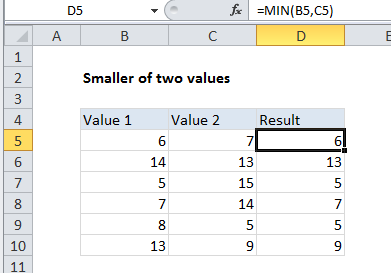## Excel Office

Excel How Tos, Tutorials, Tips & Tricks, Shortcuts

# Smaller of two values in Excel

This tutorial shows how to calculate Smaller of two values in Excel using the example below;

To get the smaller, or lesser, of two values, you can use the MIN function.

## Formula

`=MIN(value1,value2)`## Explanation

In the example shown, the formula in D5 is:

`=MIN(B5,C5)`

### How this formula works

The MIN function is fully automatic – it returns the smallest value in the numbers provided. In this case, we give MIN function two values:

`=MIN(B5,C5)`

and MIN returns the smaller value.

Also See:   How to get last row in mixed data with blanks in Excel

### Elegant alternative to IF

The MIN function can be a compact and elegant replacement for the IF function. For example, the formula above can be written with IF like so:

`=IF(B5<=C5,B5,C5)`

However, the MIN version is 6 characters shorter (11 vs. 17), and contains no redundant references, so it is less prone to errors.

Also See:   9 Mathematical Computations Example using Excel Statistical Function